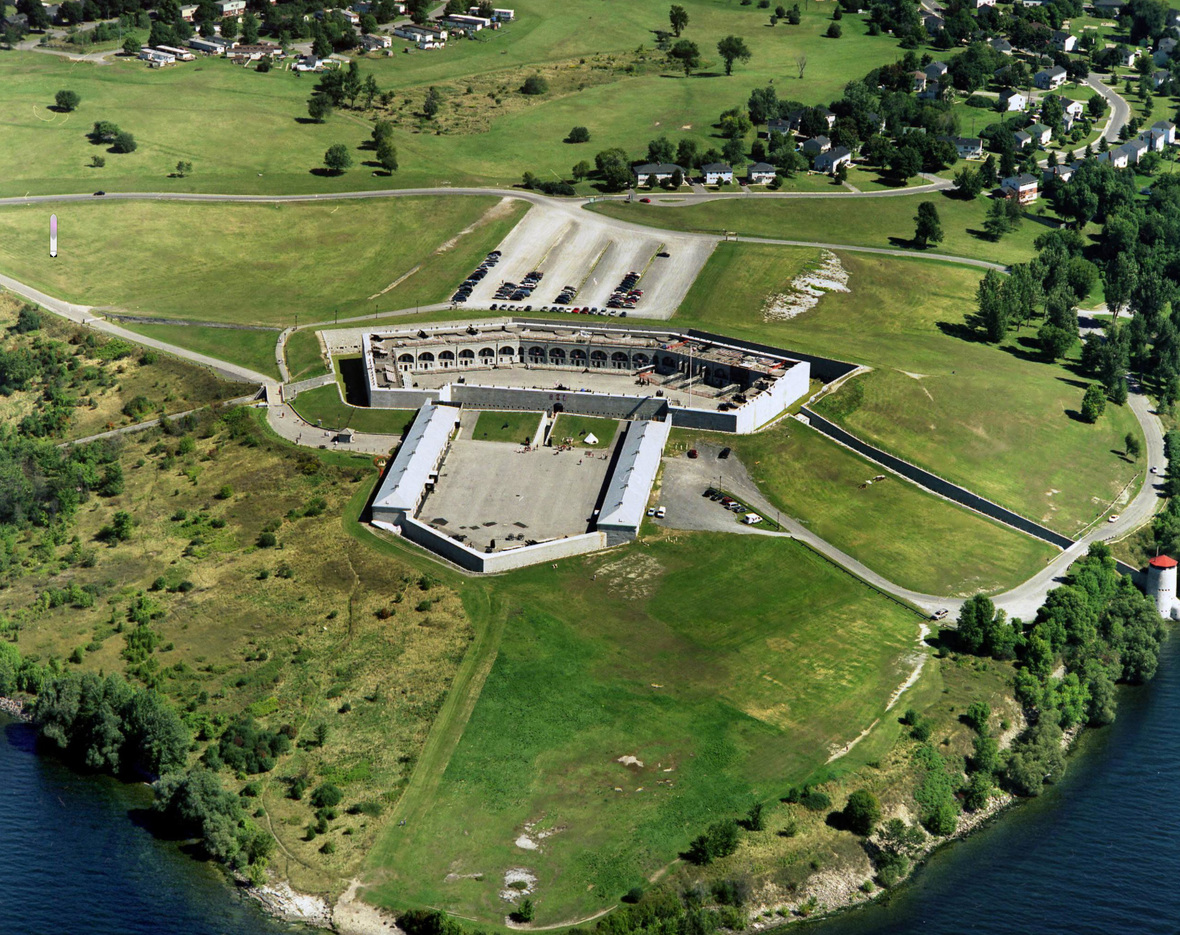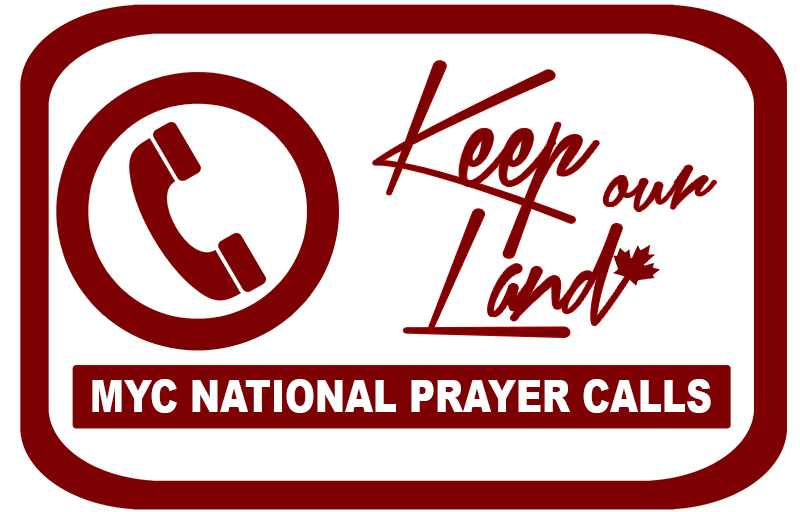Like   Tweet   Pin   +1   in
 /* styles */ We are now just a few days away from Canada's historic 150th birthday! This is a significant anniversary and there are of course many events and celebrations planned all across the nation. But we at MY Canada will be spending Canada Day in Kingston, Ontario, joining with thousands of Canadians for a full day of prayer and fasting for the nation at TheCRY Kingston. We believe that this is going to be an historic day and are full of faith for all that God wants to do. We would be thrilled if you could join us in Kingston this Saturday!
 table div table+table+table div table,table.module-2{width:50.94%;float:left;padding:0}table div table+table+table div table a{border:0 none;text-decoration:none}table div table+table+table div table img{width:100%!important;border:0 none;text-decoration:none}table div table+table+table div table td{width:100%;padding:0 20px 20px 0}/* styles */ On Friday June 23rd, MY Canada's National Director, Faytene Grasseschi, did a Facebook Live video sharing some exciting updates regarding TheCRY Kingston. Click here or on the image to the left to watch now.
 /* styles */ Please click below to register now, or scroll down to learn more about TheCRY Kingston including how you can help partner in the gathering through prayer and/or giving.
 table div table+table+table+table+table div table td,table.module-4{width:100%;padding:0}table div table+table+table+table+table div table{width:100%;float:none;margin-left:auto;margin-right:auto;padding:0}table div table+table+table+table+table div table a{border:0 none;text-decoration:none}table div table+table+table+table+table div table img{width:100%!important;border:0 none;text-decoration:none}/* styles */

## SCHEDULE:

Friday, June 30th – Pre-CRY Rally

Time: 7pm.
Location: St. Paul the Apostle Church, 1111 Taylor Kidd Blvd, Kingston, ON, K7M 8G8

Friday, June 30th – Saturday, July 1st – All night prayer for Canada lead by various houses of prayer & prayer groups.

Time: 10pm – 8am
Location: 999 Sydenham Road, Kingston, ON K7M 3L8 (Third Day Worship Centre)
Notice: Camping is available on-site on Friday night (field behind the church) for those who have tents or campers.

Saturday, July 1st – TheCRY Solemn Assembly

Time: 9am – Dusk (Ending with July 1st Fireworks) // No breaks.
Location: Front Field (Hill), Fort Henry, Kingston, ON

YES TV and 100 Huntley Street will be broadcasting live from TheCRY Kingston from 8-10:30pm EST. Check your local cable company menu for the channel number in your area.

## OTHER DETAILS:

For information about hotels, where TheCRY will be located at Fort Henry (front field), accessibility access to the front field of Fort Henry, what to bring to TheCRY, where to park and other similar details please visit our Details and Registration page.

 table.module-6{width:99.43%;padding:0}table div table+table+table+table+table+table+table div table{width:99.43%;float:none;margin-left:auto;margin-right:auto;padding:0}table div table+table+table+table+table+table+table div table a{border:0 none;text-decoration:none}table div table+table+table+table+table+table+table div table img{width:100%!important;border:0 none;text-decoration:none}table div table+table+table+table+table+table+table div table td{width:100%;padding:0}/* styles */
 table div table+table+table+table+table+table+table+table div table{width:100%;padding:0}table div table+table+table+table+table+table+table+table div table table{padding:0;float:left!important;width:51.886%!important}table div table+table+table+table+table+table+table+table div table table+table td,table div table+table+table+table+table+table+table+table div table td{padding-left:0;padding-right:0}table div table+table+table+table+table+table+table+table div table table td{padding-left:0;padding-right:20px}table div table+table+table+table+table+table+table+table div table table+table{float:left!important;width:48.114%!important}/* styles */
 table div table+table+table+table+table+table+table+table+table div table{width:100%;padding:0}table div table+table+table+table+table+table+table+table+table div table table{padding:0;float:left!important;width:51.886%!important}table div table+table+table+table+table+table+table+table+table div table table+table td,table div table+table+table+table+table+table+table+table+table div table td{padding-left:0;padding-right:0}table div table+table+table+table+table+table+table+table+table div table table td{padding-left:0;padding-right:20px}table div table+table+table+table+table+table+table+table+table div table table+table{float:left!important;width:48.114%!important}/* styles */
 table div table+table+table+table+table+table+table+table+table+table div table td,table.module-9{width:100%;padding:0}table div table+table+table+table+table+table+table+table+table+table div table{width:100%;float:none;margin-left:auto;margin-right:auto;padding:0}table div table+table+table+table+table+table+table+table+table+table div table a{border:0 none;text-decoration:none}table div table+table+table+table+table+table+table+table+table+table div table img{width:100%!important;border:0 none;text-decoration:none}/* styles */
 table div table+table+table+table+table+table+table+table+table+table+table div table{width:100%;padding:0}table div table+table+table+table+table+table+table+table+table+table+table div table img{width:96.23%;padding:0;float:none}table div table+table+table+table+table+table+table+table+table+table+table div table td{width:100%;padding:0 1.88% 18px}/* styles */## A SPECIAL LOCATION

The venue for TheCRY Kingston is the historic Fort Henry.

David Mainse, one of Canada’s most respected Christian leaders and the founder of 100 Huntley Street & Crossroads Christian Communications, recently shared with us that his very own father was posted to Fort Henry (photo left) when he served in the Canadian military. Every night David’s father and a co-soldier would walk the perimeter of Fort Henry for security rounds. With each round they would choose a scripture to memorize as they walked. One would go one direction around the Fort, the other went the other direction. With each step they would speak the scripture until they met up again at which point they would recite the scripture to each other.

## Special Invites / Lorna Dueck of 100 Huntley Street, Brian Warren of the 700 Club Canada & More

 table div table+table+table+table+table+table+table+table+table+table+table+table+table+table div table td,table.module-13{width:100%;padding:0}table div table+table+table+table+table+table+table+table+table+table+table+table+table+table div table{width:100%;float:none;margin-left:auto;margin-right:auto;padding:0}table div table+table+table+table+table+table+table+table+table+table+table+table+table+table div table a{border:0 none;text-decoration:none}table div table+table+table+table+table+table+table+table+table+table+table+table+table+table div table img{width:100%!important;border:0 none;text-decoration:none}/* styles */
 table div table+table+table+table+table+table+table+table+table+table+table+table+table+table+table div table{width:100%;padding:0}table div table+table+table+table+table+table+table+table+table+table+table+table+table+table+table div table img{width:96.23%;padding:0;float:none}table div table+table+table+table+table+table+table+table+table+table+table+table+table+table+table div table td{width:100%;padding:0 1.88% 18px}/* styles */table div table+table+table+table+table+table+table+table+table+table+table+table+table+table+table+table div table td,table.module-15{width:100%;padding:0}table div table+table+table+table+table+table+table+table+table+table+table+table+table+table+table+table div table{width:100%;float:none;margin-left:auto;margin-right:auto;padding:0}table div table+table+table+table+table+table+table+table+table+table+table+table+table+table+table+table div table a{border:0 none;text-decoration:none}table div table+table+table+table+table+table+table+table+table+table+table+table+table+table+table+table div table img{width:100%!important;border:0 none;text-decoration:none}/* styles */

 table div table+table+table+table+table+table+table+table+table+table+table+table+table+table+table+table+table+table div table{width:100%;padding:0}table div table+table+table+table+table+table+table+table+table+table+table+table+table+table+table+table+table+table div table img{width:96.23%;padding:0;float:none}table div table+table+table+table+table+table+table+table+table+table+table+table+table+table+table+table+table+table div table td{width:100%;padding:0 1.88% 18px}/* styles */TheCRY Kingston will be made possible through the generous donations of believers who have a hunger to see God's purposes in Canada.

All prayer and worship leaders pay their own way and serve freely on the day. TheCRY truly is an offering to the Lord on behalf of the nation.

Will you consider sowing a strategic seed at this time for TheCRY Kingston?

In order to make TheCRY Kingston on Canada’s 150th birthday a reality we are specifically believing God for 25 churches, ministries or businesses that will sow a symbolic seed of \$1,500 for Canada’s 150th and 400 individuals to sow a symbolic seed of \$150 for Canada’s 150th.

Update:

Current Projected Budget: \$137,299.63 (Recently Updated)
Remaining Need: \$10,496.98

Any donation amount is deeply appreciated.

 table div table+table+table+table+table+table+table+table+table+table+table+table+table+table+table+table+table+table+table+table+table div table{width:100%;padding:0}table div table+table+table+table+table+table+table+table+table+table+table+table+table+table+table+table+table+table+table+table+table div table img{width:96.23%;padding:0;float:none}table div table+table+table+table+table+table+table+table+table+table+table+table+table+table+table+table+table+table+table+table+table div table td{width:100%;padding:0 1.88% 18px}/* styles *//* styles */ We would be so grateful if you would help us spread the word regarding TheCRY Kingston! Together we are so much stronger and can do so much more. For a variety of ways that you can help share about TheCRY Kingston with your friends please click here.
 table div table+table+table+table+table+table+table+table+table+table+table+table+table+table+table+table+table+table+table+table+table+table+table+table div table{width:100%;padding:0}table div table+table+table+table+table+table+table+table+table+table+table+table+table+table+table+table+table+table+table+table+table+table+table+table div table img{width:96.23%;padding:0;float:none}table div table+table+table+table+table+table+table+table+table+table+table+table+table+table+table+table+table+table+table+table+table+table+table+table div table td{width:100%;padding:0 1.88% 18px}/* styles */## ANNOUNCEMENTS:

 table div table+table+table+table+table+table+table+table+table+table+table+table+table+table+table+table+table+table+table+table+table+table+table+table+table+table div table{width:100%;padding:0}table div table+table+table+table+table+table+table+table+table+table+table+table+table+table+table+table+table+table+table+table+table+table+table+table+table+table div table img{width:96.23%;padding:0;float:none}table div table+table+table+table+table+table+table+table+table+table+table+table+table+table+table+table+table+table+table+table+table+table+table+table+table+table div table td{width:100%;padding:0 1.88% 18px}/* styles */MY Canada is hosting national prayer conference calls every second Tuesday at noon EST.

The next call is July 4th, 2017.

 table div table+table+table+table+table+table+table+table+table+table+table+table+table+table+table+table+table+table+table+table+table+table+table+table+table+table+table+table+table div table{width:100%;padding:0}table div table+table+table+table+table+table+table+table+table+table+table+table+table+table+table+table+table+table+table+table+table+table+table+table+table+table+table+table+table div table img{width:96.23%;padding:0;float:none}table div table+table+table+table+table+table+table+table+table+table+table+table+table+table+table+table+table+table+table+table+table+table+table+table+table+table+table+table+table div table td{width:100%;padding:0 1.88% 18px}/* styles */## MEMORIAL FOR THE UNBORN IN CANADA

 table div table+table+table+table+table+table+table+table+table+table+table+table+table+table+table+table+table+table+table+table+table+table+table+table+table+table+table+table+table+table+table div table td,table.module-30{width:100%;padding:0}table div table+table+table+table+table+table+table+table+table+table+table+table+table+table+table+table+table+table+table+table+table+table+table+table+table+table+table+table+table+table+table div table{width:100%;float:none;margin-left:auto;margin-right:auto;padding:0}table div table+table+table+table+table+table+table+table+table+table+table+table+table+table+table+table+table+table+table+table+table+table+table+table+table+table+table+table+table+table+table div table a{border:0 none;text-decoration:none}table div table+table+table+table+table+table+table+table+table+table+table+table+table+table+table+table+table+table+table+table+table+table+table+table+table+table+table+table+table+table+table div table img{width:100%!important;border:0 none;text-decoration:none}/* styles */
 table div table+table+table+table+table+table+table+table+table+table+table+table+table+table+table+table+table+table+table+table+table+table+table+table+table+table+table+table+table+table+table+table+table div table{width:100%;padding:0}table div table+table+table+table+table+table+table+table+table+table+table+table+table+table+table+table+table+table+table+table+table+table+table+table+table+table+table+table+table+table+table+table+table div table img{width:96.23%;padding:0;float:none}table div table+table+table+table+table+table+table+table+table+table+table+table+table+table+table+table+table+table+table+table+table+table+table+table+table+table+table+table+table+table+table+table+table div table td{width:100%;padding:0 1.88% 18px}/* styles */